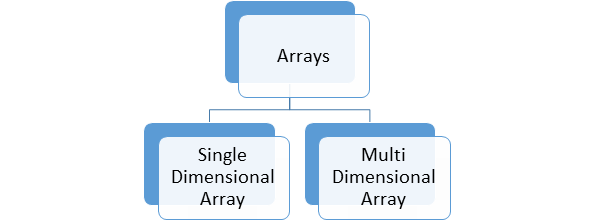# What are Arrays?

• An array is a collection of similar elements of same data type at a contiguous memory location. The array is a data structure which stores the fixed size of elements in sequence.
• The size and the data type of an array cannot be changed after the declaration. The length of the array is defined when the array is created and the length of the array is fixed it cannot be changed.
• In arrays, each value is an element and these elements are accessed through an index.

0             1            2             3             4              5

In the above figure the array length is 6 and it always begins with index 0.

# How to declare arrays?

To declare the arrays you need to specify the data type and the number of elements.

# Let us have a look at the syntax:

type array_name[array_size];

int marks;

# How to initialize arrays?

In C++ the arrays can be initialized by giving a single statement.

# Like this

int marks = {67, 82, 95, 37, 50};

• The values cannot be larger than the number of elements that we declare for the array between square brackets[]. If you do not provide the size of an array it will calculate the size of the array by providing the values while initializing.

# Let us have a look at the example:

int marks [ ] = {45,98,56,70,85};

• So, from the above example you can see we have not declared the size of an array so, on the basis of the values the array will calculate the size and will allocate the memory. But if we try to add any one more value extra in the array it will show an error.

• It is easy to sort the data in arrays by using the swapping technique.
• You can randomly access the values of array with the help of array index.
• It provides the code optimization that means less code is required.
• You can retrieve the data from an array easily.

• The main disadvantage of the arrays is the size limit. In this, you can store the fixed size of elements.

# Accessing Arrays

• The array elements are accessed by indexing the array name and it can be done by putting the index of the element within the square brackets after the name of the array.

# Let us have a look at the example:

int  marks = value;

• From the above example you can see that the array will provide the 5th element from the array and will be provided to the marks variable.
 45 98 56 70 85

marks    marks  marks   marks   marks

• So, from the above representation you can see that the array will provide the 85 to the marks variable.

# Types of Array# Single Dimensional Array

• The single dimensional array is also known as one dimensional array which is having a group of elements having the same datatype and same with same name.

# Let us have a look at the syntax:

datatype array_name[array_size];

# Multidimensional Array

• The multi-dimensional array is that array in which data is arranged in the form of array of arrays. The multi-dimensional array can have as many dimensions as it required.
• So, two dimensional and three dimensional arrays are commonly used.

# Let us have a look at the syntax:

datatype array_name [a1][a2][a3]…[an];

• In this, the array_name is the array of type datatype and it has n dimensions. The number of elements in multi-dimensional array is same as the product size of all the dimensions or you can say the total number of element in array_name is a1*a2*a3*…an.